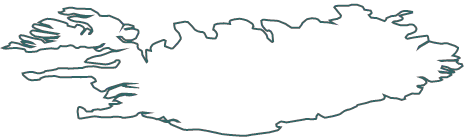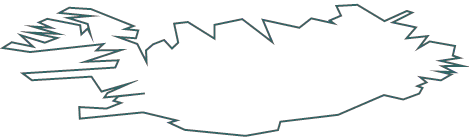﻿ Map Simplification
Nevron .NET Vision
Diagram for .NET / User's Guide / Maps / Map Simplification

In This Topic
Map Simplification
In This Topic

Simplification of a shape is a process which involves removing of points from the shape's outline. The algorithm tries to preserve the original contour as much as possible. To control the level of data point reduction you specify a simplification factor - the greater the factor, the greater the reduction. The algorithm interprets the simplification factor as follows: is p and q are 2 non adjacent points from the list and all points between p and q in the list are located at a distance smaller than the simplification factor from the line segment p-q then all points between p and q are removed. When working with maps you can simplify the boundaries of a shape in the OnPolygonCreated method of the feature created callback:

C#
Copy Code
```NPolygonShape polygonShape = (NPolygonShape)element;
NPointFList simplifiedPointList = NPointFList.Simplify(polygonShape.Points, 3);
polygonShape.Points = simplifiedPointList.ToArray();
```
Visual Basic
Copy Code
```Dim polygonShape As NPolygonShape = (NPolygonShape)element
Dim simplifiedPointList As NPointFList = NPointFList.Simplify(polygonShape.Points, 3)
polygonShape.Points = simplifiedPointList.ToArray()
```Fig. 1: The country of Iceland - original geographical data (1084 points)Fig. 2: The country of Iceland - after the simplification (103 points)

By reducing the number of points, the electronic size of the map is reduced, and this can lead to an improvement in map display performance and the storage capacity needed for saving the map.